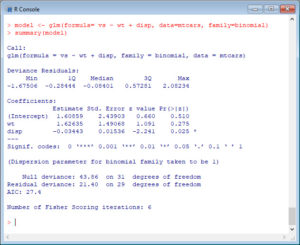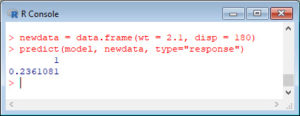In Part 15, let’s see how to create simple Generalised Linear Models in R.
Ordinary Least Squares regression provides linear models of continuous variables. However, much data of interest to statisticians and researchers are not continuous and so other methods must be used to create useful predictive models. The `glm()` command is designed to perform generalised linear models (regressions) on binary outcome data, count data, probability data, proportion data and many other data types. In this posting we explore the use of R’s `glm()` command on such data types. Let’s take a look at a simple example where we model binary data.
In the `mtcars` data set, the variable `vs` indicates if a car has a V engine or a straight engine.
We want to create a model that helps us to estimate the probability of a vehicle having a V engine or a straight engine given a weight of 2100 lbs and engine displacement of 180 cubic inches. We use the `glm()` function, include the variables in the usual way, and specify a binomial error distribution, as follows:
```model <- glm(formula= vs ~ wt + disp, data=mtcars, family=binomial) summary(model)```We see that weight influences vs positively, while displacement has a slightly negative effect.
The model output is somewhat different from that of an ordinary least squares model. I will explain the output in more detail in the next blog, but for now, let’s continue with our calculations.
We create a data frame called `newdata`, in which we include the desired values for our prediction.
`newdata = data.frame(wt = 2.1, disp = 180)`
Now we use the `predict()` function to calculate the probability. We include the argument `type="response"` in order to get our prediction.
`predict(model, newdata, type="response")`The predicted probability is 0.24.
That wasn’t so hard! In Blog 16 I will explain more about the output we got from the `glm()` function.
See you later!
David

#### Annex: R codes used

```# Estimate Generalised Linear Model and display results.
model <- glm(formula= vs ~ wt + disp, data=mtcars, family=binomial)
summary(model)

# Create extended data frame with a new value.
newdata = data.frame(wt = 2.1, disp = 180)

# Prediction of probability based on estimated model.
predict(model, newdata, type="response")
```